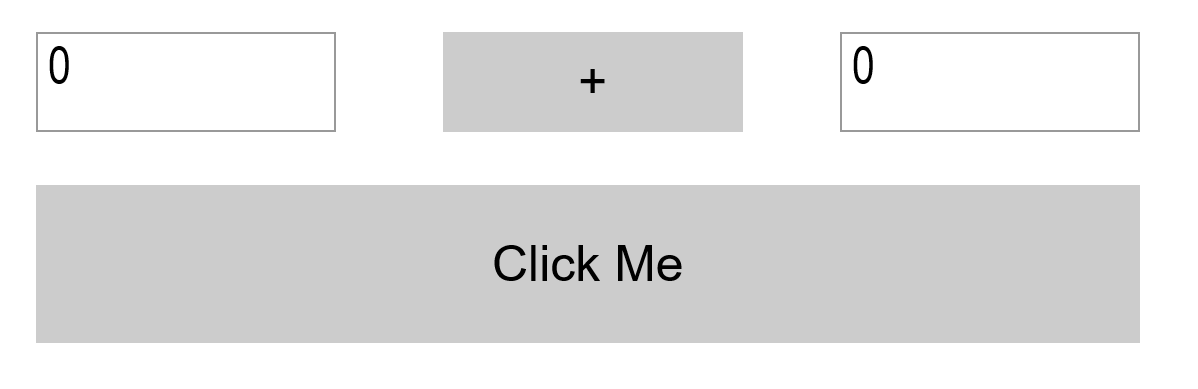# Calculator Script Example

In this scripting example the structure of a very simple calculator is explained, which can add and subtract.The numbers to be calculated are entered in the left and right text fields. Using the button which is currently set to “+”, the type of calculation can be determined:

``````if screens['Screen1'].PlusMinus.text == '+' then
screens['Screen1'].PlusMinus.text = '-'
else
screens['Screen1'].PlusMinus.text = '+'
end

``````

Therefore it is checked which text is monentan in the button (which PlusMinus is called). If the text is ‘+’, it is set to ‘-‘. If the text is not ‘+’ it must be ‘-‘, so it is set to’+’ without checking.

Click the “Click me” button to start the calculation:

``````local result = 0
--Check the text in the plus minus button

if screens['Screen1'].PlusMinus.text == '+' then
result = tonumber(screens['Screen1'].Left.text) + tonumber(screens['Screen1'].Right.text)
else
result = tonumber(screens['Screen1'].Left.text) - tonumber(screens['Screen1'].Right.text)
end

-- Show the result in a dialog
screens['Screen1'].showdialog('Result', result)

``````

First a local variable is created for the result `local result`. Then the PlusMinus button is used to determine whether the numbers in the two text fields should be added or subtracted. The result of this calculation in the script is then written to the local variable ‘result’ and last displayed on the screen via `screens['Screen1'].showdialog('Result', result)` As pop-up notification

This example can be found in the Peakboard Designer under Templates -> Scripting, or can be downloaded via this Link.